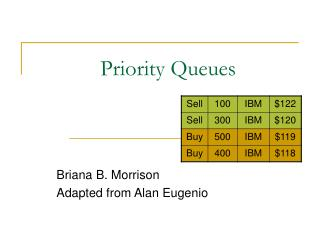DownloadDownload PresentationPriority Queues

# Priority Queues

Télécharger la présentation## Priority Queues

- - - - - - - - - - - - - - - - - - - - - - - - - - - E N D - - - - - - - - - - - - - - - - - - - - - - - - - - -
##### Presentation Transcript

1. Priority Queues Briana B. Morrison Adapted from Alan Eugenio

2. Topics • API • Application • Implementation Priority Queues

3. Priority Queue A Special form of queue from which items are removed according to their designated priority and not the order in which they entered. Items entered the queue in sequential order but will be removed in the order #2, #1, #4, #3. Priority Queues

4. A PRIORITY QUEUE IS A CONTAINER IN WHICH ACCESS OR DELETION IS OF THE HIGHEST-PRIORITY ITEM, ACCORDING TO SOME WAY OF ASSIGNING PRIORITIES TO ITEMS. Priority Queues

5. Priority Queues

6. Priority Queues

7. Priority Queues

8. Priority Queues

9. Priority Queues

10. Priority Queues

11. Priority Queues

12. Priority Queues

13. Priority Queues

14. Priority Queues

15. Priority Queues

16. Priority Queues

17. Priority Queues

18. Priority Queues

19. Priority Queues

20. Priority Queues

21. Priority Queues

22. Priority Queues

23. priority_queue(); Create an empty priority queue. Type T must implement the operator <. CLASS priority_queue CLASS priority_queue <queue> <queue> Constructor Operations bool empty() const; Check whether the priority queue is empty. Return true if it is empty, and false otherwise. Create void pop(); Remove the item of highest priority from the queue. Precondition: The priority queue is not empty. Postcondition: The priority queue has 1 less element Priority Queues

24. CLASS priority_queue <queue> Operations void push(const T& item); Insert the argument item into the priority queue. Postcondition: The priority queue contains a new element. int size() const; Return the number of items in the priority queue. T& top(); Return a reference to the item having the highest priority. Precondition: The priority queue is not empty. const T& top(); Constant version of top(). Priority Queues

25. A comparator encapsulates the action of comparing two objects according to a given total order relation A generic priority queue uses a comparator as a template argument, to define the comparison function (<,=,>) The comparator is external to the keys being compared. Thus, the same objects can be sorted in different ways by using different comparators. When the priority queue needs to compare two keys, it uses its comparator Comparator ADT Priority Queues

26. A comparator class overloads the “()” operator with a comparison function. Example: Compare two points in the plane lexicographically.class LexCompare {public:int operator()(Point a, Point b) { if (a.x < b.x) return –1 else if (a.x > b.x) return +1 else if (a.y < b.y) return –1 else if (a.y > b.y) return +1 else return 0; }}; Using Comparators in C++ To use the comparator, define an object of this type, and invoke it using its “()” operator: Example of usage:Point p(2.3, 4.5);Point q(1.7, 7.3);LexCompare lexCompare;if (lexCompare(p, q) < 0) cout << “p less than q”;else if (lexCompare(p, q) == 0) cout << “p equals q”;else if (lexCompare(p, q) > 0) cout << “p greater than q”; Priority Queues

27. Applications • Applications: • Standby flyers • Auctions • Stock market • Sorting • Huffman Coding Priority Queues

28. Sorting with a Priority Queue • We can use a priority queue to sort a set of comparable elements • Insert the elements one by one with a series of push(e) operations • Remove the elements in sorted order with a series of pop() operations • The running time of this sorting method depends on the priority queue implementation AlgorithmPQ-Sort(S, C) • Inputsequence S, comparator C for the elements of S • Outputsequence S sorted in increasing order according to C P priority queue with comparator C while!S.empty () e S.remove (S.first ()) P.push(e) while!P.empty() e P.top()P.pop() S.insertLast(e) Priority Queues

29. Priority Queues

30. Priority Queues

31. Priority Queues

32. Priority Queues

33. Priority Queues

34. Priority Queues

35. Priority Queues

36. Priority Queues

37. Priority Queues

38. Priority Queues

39. Priority Queues

40. Priority Queues

41. Priority Queues

42. Priority Queues

43. Priority Queues

44. Priority Queues

45. Priority Queues

46. Priority Queues

47. Priority Queues

48. Priority Queues

49. Priority Queues

50. Huffman Trees Problem Input: A set of symbols, each with a frequency of occurrence. Desired output: A Huffman tree giving a code that minimizes the bit length of strings consisting of those symbols with that frequency of occurrence. Strategy: Starting with single-symbol trees, repeatedly combine the two lowest-frequency trees, giving one new tree of frequency = sum of the two frequencies. Stop when we have a single tree. Priority Queues